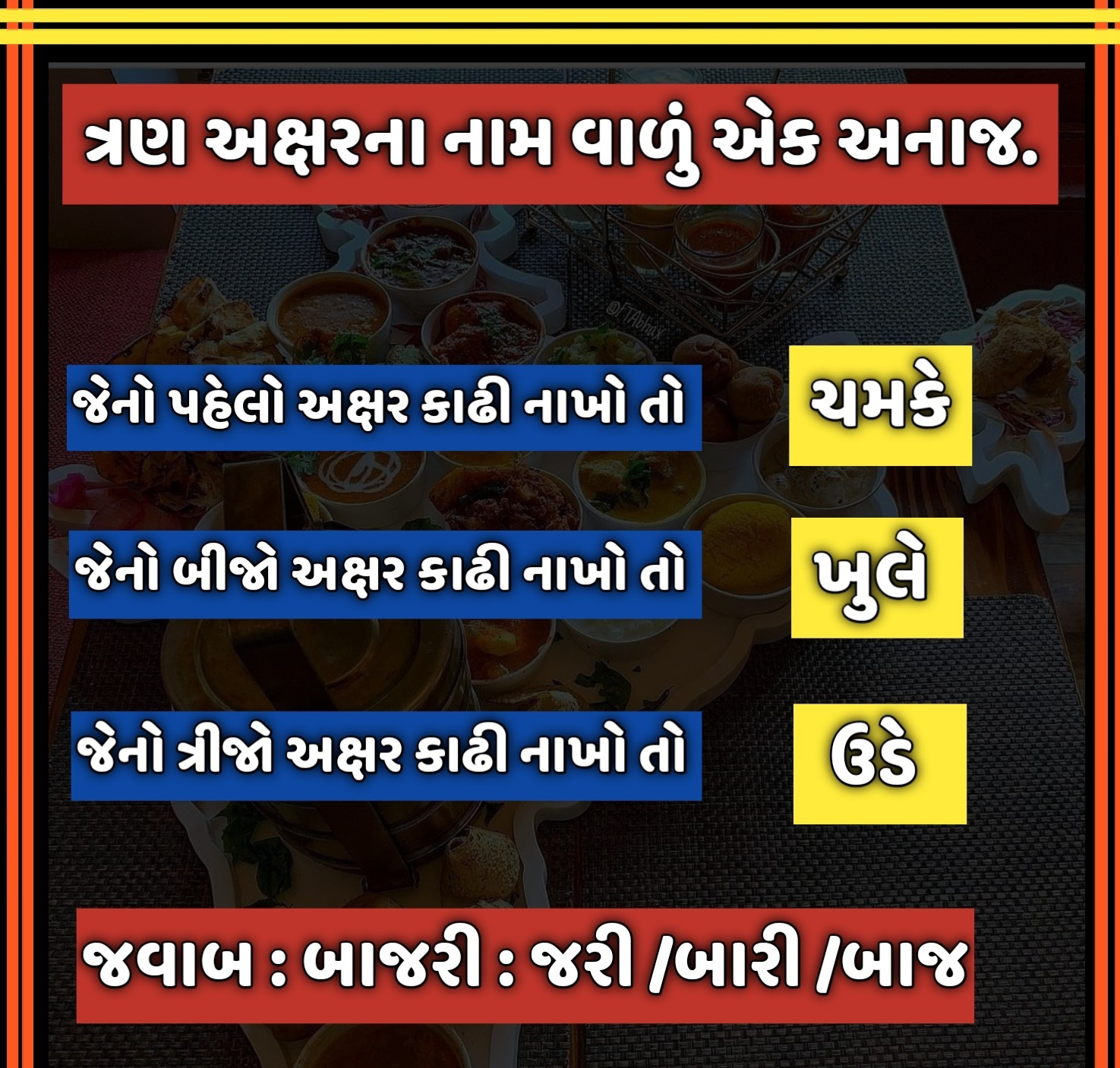## Sunday, 29 August 2021

### Puzzles Riddles Challenging Brainstorming game for testing your math skills

Math Puzzles Riddles Challenging Brainstorming game for testing your math skills

This Math Games Puzzles Riddles game 2020 has 100 interesting logical maths brain teasers with hints and answers with solutions to improve your mathematics.

100 Free Cool Math Games Puzzles & Riddles Brain Teasers 2020

If you'd like exercising and challenging your brain with mathematics, then this numbrix Math Puzzles Game is for you. This game has 100 maths riddles and brain teasers which require mathematics to solve this like, math equations, math patterns, reasoning, algebra, arithmetic puzzle, black math logic puzzles, etc. These riddles are simple to hard when the level increase which will let you think out of the box.

Math Puzzles tests your IQ level with mathematical riddles. Challenge yourself with different levels of black math puzzles and stretch the limits of your intelligence. Each Math Game is prepared with an approach of an IQ test. Improve your mathematics, challenge your brain with this game. This is a brainstorming game. There are many hard levels which you may not have solved then you can take help which provides you hints, skip a level and solutions.This cool math game increases your mental thinking and analytical level. This game is for both adults and child . This is a free puzzle riddle game. Here you find interesting Megamind logical puzzles, classical riddles.

Math games help to develop mental skills, improve memory, focus, and brain speed. Keep your brain fit through regular math exercises.

Our games are ideal for adults and child who like to solve interesting puzzles and problems.

Mathematical Formula used
:- Multipication
:- Substract
:- Divide
:- Mudulus
:- Square
:- CubeGame Features
These are the features that make Math Puzzle & Riddle Games the best game to help you to do a great mental brainstorming and logical math exercise.

:- 100 Levels to test your brain and increase your mathematical ability.
:- Use hints to think in the right direction.
:- Maths game is for kids as well as adults.
:- Find the solutions with the correct answer to the questions
:- Skip Level when you want to skip.
:- Reset the maths game whenever complete game. Solve these math questions again and again and keep improving at solving these.
:- User-friendly User Interface
:- This game is free with ads. If you want to remove ads you should buy the pro version